# Review — Colorful Image Colorization (Self-Supervised Learning)

## Colorization as Pretext Task in Self-Supervised Learning, Outperforms Context Prediction & Context Encoders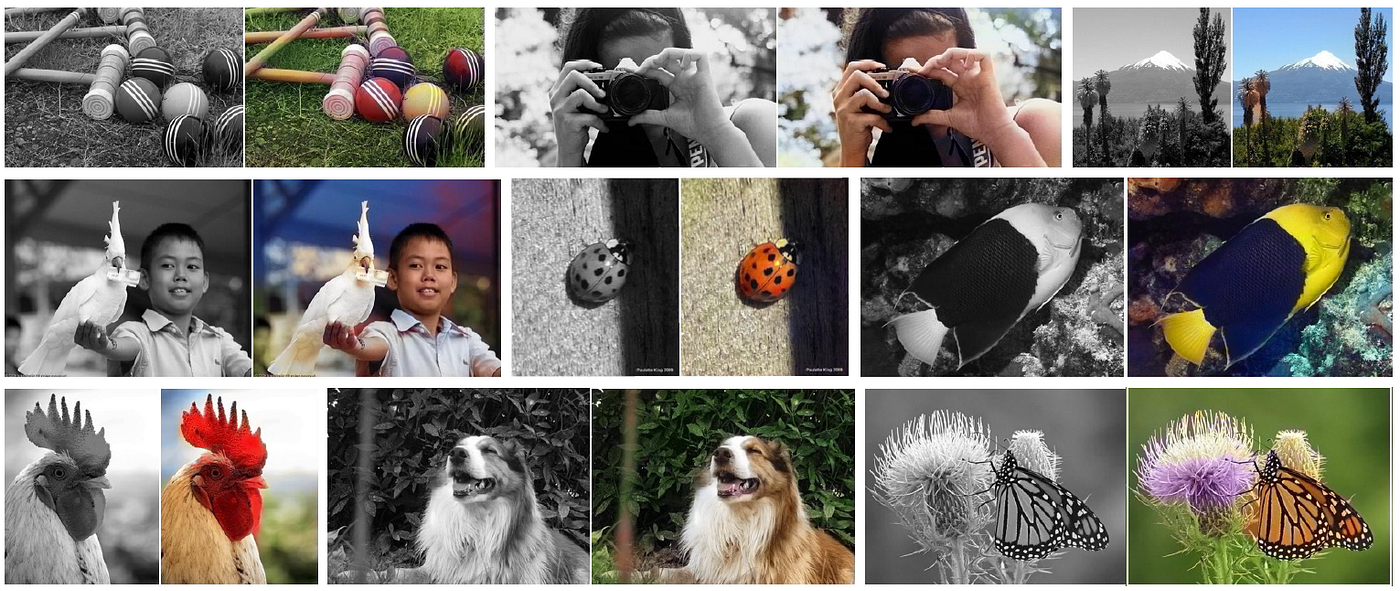Example Input Grayscale Photos and Output Colorizations

In this story, Colorful Image Colorization, by University of California, Berkeley, is reviewed. In this paper:

• A fully automatic approach is designed, which produces vibrant and realistic colorizations for a grayscale image.
• This proposed colorization task is treated as a powerful pretext task for self-supervised feature learning, acting as a cross-channel encoder.

It is a paper in 2016 ECCV with over 1900 citations. (Sik-Ho Tsang @ Medium)

# Outline

1. Colorful Image Colorization
2. Colorization Results
3. Self-Supervised Learning Results

# 1. Colorful Image Colorization

• The color space used is CIE Lab color space.
• Given an input lightness channel X (L), the objective is to learn a mapping to the two associated color channels Y (ab).
• Each conv layer refers to a block of 2 or 3 repeated conv and ReLU layers, followed by a BatchNorm layer. The net has no pool layers, only spatial downsampling or upsampling is used between conv blocks if needed.

## 1.1. L2 Loss is NOT Robust

• Using naïve L2 loss as shown below is not robust to the inherent ambiguity and multimodal nature of the colorization problem:
• For example:
1. if an object can take on a set of distinct ab values, the optimal solution to the Euclidean loss will be the mean of the set. This averaging effect favors grayish, desaturated results.
2. Additionally, if the set of plausible colorizations is non-convex, the solution will in fact be out of the set, giving implausible results.

## 1.2. Multinomial Classification Loss

• The problem is treated as multinomial classification.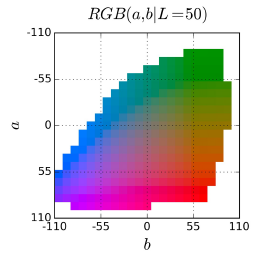Quantized ab color space with a grid size of 10
• The ab output space is quantized into bins with grid size 10 and keep the Q = 313 values which are in-gamut, as above.
• For a given input X, we learn a mapping ^Z= G(X) to a probability distribution over possible colors:
• where Q is the number of quantized ab values.
• Z is the vector converted from the ground truth color Y, using a soft-encoding scheme:
• The 5-nearest neighbors to Y_h,w in the output space are selected and weighted proportionally to their distance from the ground truth using a Gaussian kernel with σ=5.
• Thus, the multinomial cross entropy loss is:
• where v(.) is a weighting term that can be used to rebalance the loss based on color-class rarity:
• Each pixel is weighed by factor w based on its closest ab bin. (λ=1/2)
• The reason to reweight is that the distribution of ab values in natural images is strongly biased towards values with low ab values, due to the appearance of backgrounds such as clouds, pavement, dirt, and walls.
• The ground truth using loss function is dominated by desaturated ab values.

To be brief, the class-imbalance problem is achieved by reweighting the loss of each pixel at train time based on the pixel color rarity as above.

• Finally, we map probability distribution ^Z to color values ^Y with function:
• This mapping H is mentioned as below in Section 1.3.

## 1.3. Class Probabilities to Point Estimates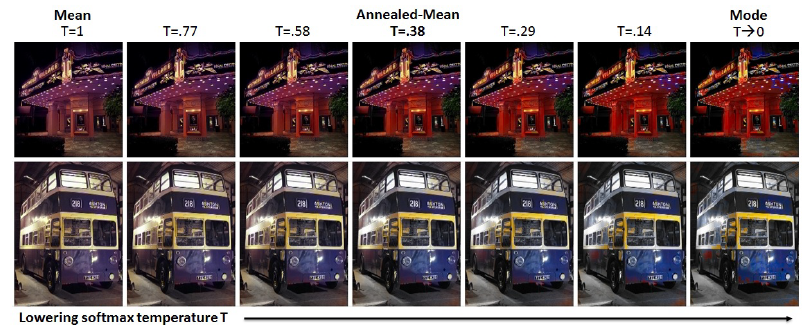e ect of temperature parameter T on the annealed-mean output
• H is defined to map the predicted distribution ^Z to point estimate ^Y in ab space:
• T=0: One choice is to take the mode of the predicted distribution for each pixel. But this provides a vibrant but sometimes spatially inconsistent result.
• T=1: On the other hand, taking the mean of the predicted distribution produces spatially consistent but desaturated results.
• The temperature T=0.38, shown in the middle column of the above figure, captures the vibrancy of the mode while maintaining the spatial coherence of the mean.
• (The introduction of temperature T into Softmax can be referenced from Model Distillation)

Hence, the final system F is the composition of CNN G, which produces a predicted distribution over all pixels, and the annealed-mean operation H, which produces a final prediction. (The system is NOT quite end-to-end trainable.)

# 2. Colorization Results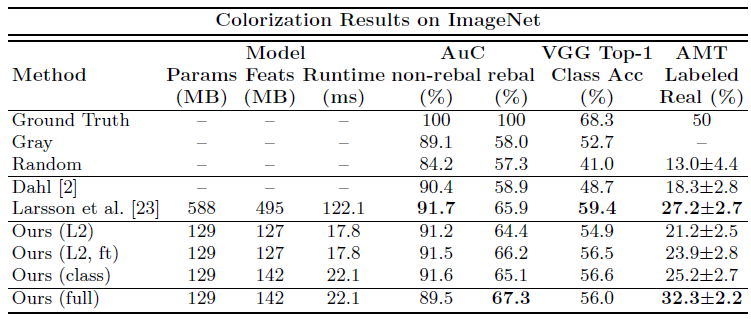Colorization results on 10k images in the ImageNet validation set
• The 1.3M images from the ImageNet training set are used for training, the first 10k images in the ImageNet validation set are used for validation, and a separate 10k images in the validation set are used for testing.
• Ours (full): Proposed network with classification loss and class rebalancing.
• Ours (class): Proposed network with classification loss only, no class rebalancing. (λ=1)
• Ours (L2): Proposed network with L2 regression loss, trained from scratch.
• Ours (L2, ft): Proposed network with L2 regression loss, fine-tuned from full classification with rebalancing network.

## 2.1. Perceptual realism (AMT)

• A real vs. fake two-alternative forced choice experiment is ran on Amazon Mechanical Turk (AMT). 40 Participants were asked to click on the photo they believed contained fake colors.

The proposed full algorithm fooled participants on 32% of trials, which is significantly higher than all compared algorithms. These results validate the effectiveness of using both a classification loss and class-rebalancing.

## 2.2. Semantic Interpretability (VGGClassification)

• It is tested by feeding our fake colorized images to a VGG. If the classifier performs well, that means the colorizations are accurate.

Classifier performance drops from 68.3% to 52.7% after ablating colors from the input. After re-colorizing using our full method, the performance is improved to 56.0%.

## 2.3. Legacy Black and White Photos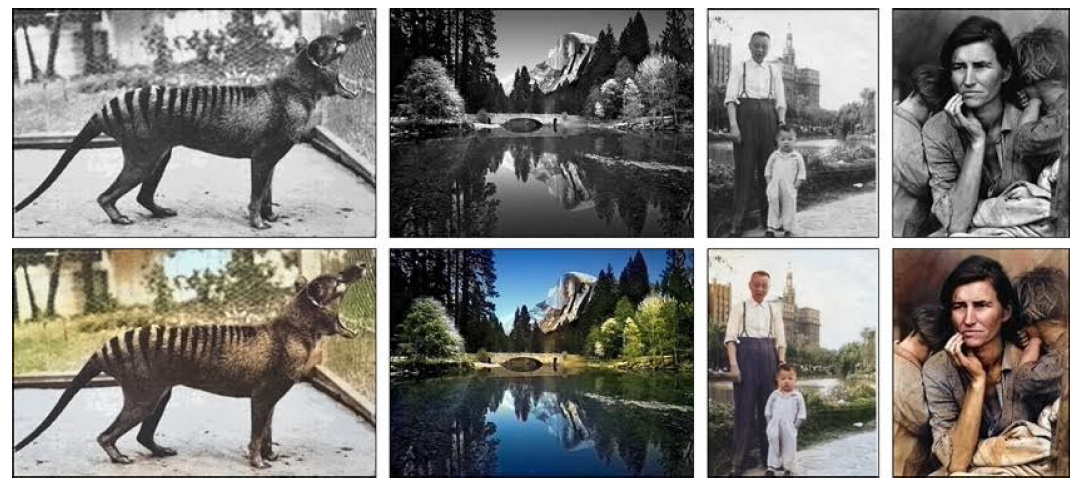Applying the proposed method to legacy black and white photos

The proposed model is still able to produce good colorizations, even though the low-level image statistics of the legacy photographs are quite different from those of the modern-day photos.

## 2.4. More Examples

• More examples and results are in the appendix of the paper.

# 3. Self-Supervised Learning Results

• The colorization approach serves as a pretext task for representation learning.
• The network model is akin to an autoencoder, except that the input and output are different image channels, suggesting the term cross-channel encoder.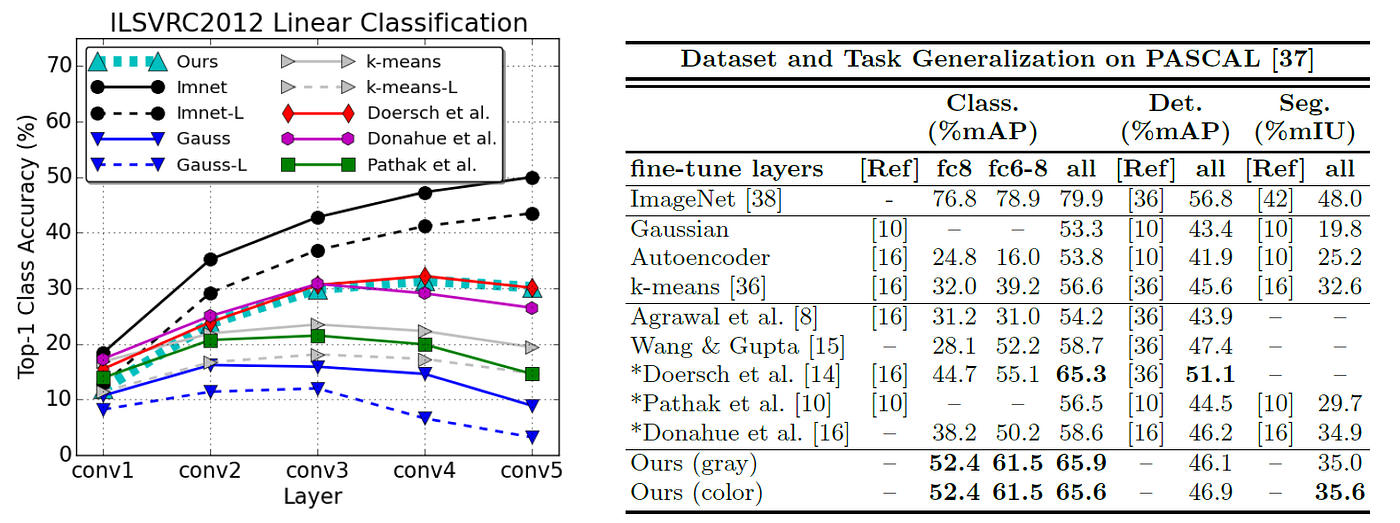Left: ImageNet Linear Classification, Right: PASCAL Tests

## 3.1. ImageNet Classification

• The pre-trained networks are frozen and linear classifiers are learnt on top of the each convolutional layer for ImageNet classification.
• Pretraining are performed without semantic label information.
• AlexNet directly trained on ImageNet classification achieves the highest performance, and serves as the ceiling for this test.
• The proposed method outperforms Gaussian, k-means, and Context Encoders .

Solving the colorization task encourages representations that linearly separate semantic classes in the trained data distribution.

## 3.2. PASCAL Classification

• The network is trained by freezing the representation up to certain points, and fine-tuning the remainder.
• The network is effectively only able to interpret grayscale images.

## 3.4. PASCAL Segmentation

• FCN architecture is used.
• The proposed grayscale fine-tuned network achieves performance of 35.0%, approximately equal to Donahue et al. , and adding in color information increases performance to 35.6%.

By learning the colorization as pretext task without ground truth labels, useful features are learnt, which can be used for downstream tasks, such as image classification, detection, and segmentation.

## Reference

[2016 ECCV] [Colorization]
Colorful Image Colorization

## Self-Supervised Learning

2008–2010 [Stacked Denoising Autoencoders] 2014 [Exemplar-CNN] 2015 [Context Prediction] 2016 [Context Encoders] [Colorization] 2017 [L³-Net] [Split-Brain Auto]

--

--